# Triangle - 8th grade (13y) - math problems

#### Number of problems found: 671

• Medians in RT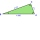The rectangular triangle ABC has a length of 10 cm and 24 cm. Points P, Q, R are the centers of the sides of this triangle. The perimeter of the PQR triangle is:
• Find the 9Find the missing angle in the triangle and then name triangle. Angles are: 95, 2x+15, x+3
• Right triangleA right triangle ABC is given, c is a hypotenuse. Find the length of the sides a, b, the angle beta if c = 5 and angle alfa = A = 35 degrees.
• TriangleCalculate the sides of the triangle if its area S = 630 and the second cathethus is shorter by 17.
• Similar trianglesIn the triangle DEF is DE = 21cm, EF = 14.7cm, DF = 28cm. The triangle D´E´F´ is similar to the triangle DEF. Calculate the lengths of the sides of the triangle D´E´F´ if the similarity coefficient is one-seventh.
• Sides od triangleSides of the triangle ABC has length 4 cm, 5 cm and 7 cm. Construct triangle A'B'C' that are similar to triangle ABC which has a circumference of 12 cm.
• Sides of triangleTriangle has circumference 42 cm. Side a is 2 times shorter than side b and sice c is 2 cm longer than side a. Determine the sizes of sides of a triangle.
• EQL triangle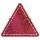Calculate inradius and circumradius of equilateral triangle with side a=77 cm.
• The sides 3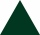The sides of an equilateral triangle are 9.4 cm, correct to the nearest one decimal place. Work out the upper bound of the side of this triangle.
• Chord of triangle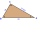If the whole chord of the triangle is 14.4 cm long, how do you calculate the shorter and longer part?
• Equilateral triangleCalculate the area of an equilateral triangle with circumference 72cm.
• Isosceles right triangleArea of an isosceles right triangle is 18 dm2. Calculate the length of its base.
• Angles of the triangleABC is a triangle. The size of the angles alpha, beta are in a ratio 4: 7. The angle gamma is greater than the angle alpha by a quarter of a straight angle. Determine angles of the triangle ABC.
• Is right-angled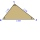Can a triangle with the sides of sqrt 3, sqrt 5 and sqrt 8 (√3, √5 and √8) be a right triangle?
• Triangle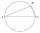Calculate the area of right triangle ΔABC, if one leg is long 14 and its opposite angle is 59°.
• Triangle anglesThe angles α, β, γ in triangle ABC are in the ratio 6:2:6. Calculate size of angles.
• Type of triangle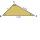How do I find the triangle type if the angle ratio is 2:3:7 ?
• Equilateral triangle v2Equilateral triangle has a perimeter 36 dm. What is its area?
• Right triangle eq2Hypotenuse of a right triangle is 9 cm longer than one leg and 8 cm longer than the second leg. Determine the circumference and area of a triangle.
• Right isosceles triangle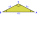What can be the area of a right isosceles triangle with a side length of 8 cm?

Do you have an interesting mathematical word problem that you can't solve it? Submit a math problem, and we can try to solve it.

We will send a solution to your e-mail address. Solved examples are also published here. Please enter the e-mail correctly and check whether you don't have a full mailbox.

Please do not submit problems from current active competitions such as Mathematical Olympiad, correspondence seminars etc...